Auto ByteScience AI# 如何使用 Highway Networks 用于语句分类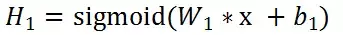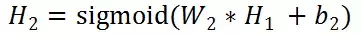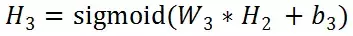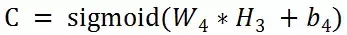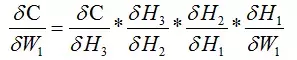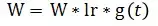1. Notation

(.) 操作代表的是矩阵按位相乘

sigmoid函数：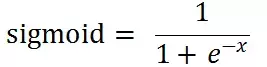2. Highway Networks formula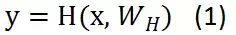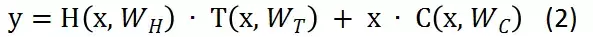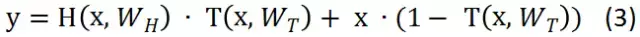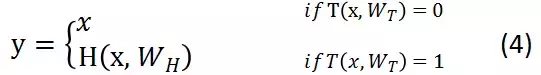1. Highway BiLSTM Networks  Structure Diagram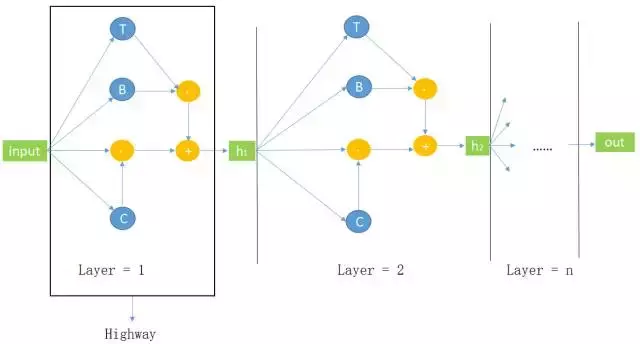2. Highway BiLSTM Networks  Demo

pytorch搭建神经网络一般需要继承nn.Module这个类，然后实现里面的forward()函数，搭建Highway BiLSTM Networks写了两个类，并使用nn.ModuleList将两个类联系起来：

``  class HBiLSTM(nn.Module):        def __init__(self, args):                super(HBiLSTM, self).__init__()                ......        def forward(self, x):                # 实现Highway BiLSTM Networks的公式                ......``
``  class HBiLSTM_model(nn.Module):         def __init__(self, args):                super(HBiLSTM_model, self).__init__()                ......                # args.layer_num_highway 代表Highway BiLSTM Networks有几层                self.highway = nn.ModuleList([HBiLSTM(args)           for _ in range(args.layer_num_highway)])                ......       def forward(self, x):                 ......                # 调用HBiLSTM类的forward()函数                for current_layer in self.highway:                    x, self.hidden = current_layer(x, self.hidden)``

``   x, hidden = self.bilstm(x, hidden)          # torch.transpose是转置操作          normal_fc = torch.transpose(x, 0, 1)``

``  source_x = source_x.contiguous()    information_source = source_x.view(source_x.size(0)                   * source_x.size(1), source_x.size(2))    information_source = self.gate_layer(information_source)    information_source = information_source.view(source_x.size(0),                   source_x.size(1), information_source.size(1))``

``  # you also can choose the strategy that zero-padding    zeros = torch.zeros(source_x.size(0), source_x.size(1),                   carry_layer.size(2) - source_x.size(2))    source_x = Variable(torch.cat((zeros, source_x.data), 2))``

``  # transformation gate layer in the formula is T    transformation_layer = F.sigmoid(information_source)    # carry gate layer in the formula is C    carry_layer = 1 - transformation_layer    # formula Y = H * T + x * C    allow_transformation = torch.mul(normal_fc, transformation_layer)    allow_carry = torch.mul(information_source, carry_layer)        information_flow = torch.add(allow_transformation, allow_carry)``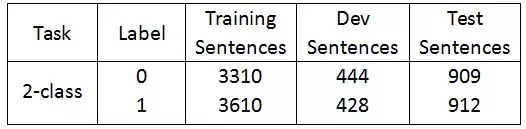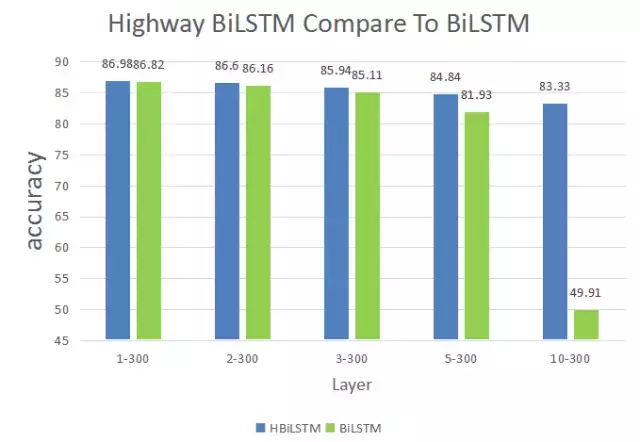References

 R. K. Srivastava, K. Greff, and J. Schmidhuber. Highway networks. arXiv:1505.00387, 2015.

 R. K. Srivastava, K. Greff, and J. Schmidhuber. Training very deep networks. 1507.06228, 2015.

 Julian Georg Zilly, Rupesh Kumar Srivastava, Jan Koutník, and Jürgen Schmidhuber. Recurrent highway networks. arXiv preprint arXiv:1607.03474, 2016.

 X. Glorot and Y. Bengio. Understanding the difficulty of training deep feedforward neural networks. In AISTATS, 2010.